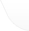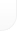热线电话: 130-5800-8007

## dedecms织梦内容页{dede:pagebreak/}分页标签

 今天在给一个织梦dedecms客户定制模板时遇到一个关于内容页 和图片集织梦dedecms分页过多 导致布局不美观的问题！ 经过一翻修改 终于搞定！ 让织梦dedecms内容和图集分页和列表页分页一样展示： 具体修改方法如下 首先找到：include/arc.archives.class.php 并且打开。 第一步查找以下代码 function GetPagebreakDM(\$totalPage,\$nowPage,\$aid)    然后从这句代码往下查看 找到  for(\$i=1;\$i<=\$totalPage;\$i++) 替换为以下代码 \$total_list=10;      if(\$nowPage >= \$total_list) {              \$i = \$nowPage-4;              \$total_list = \$nowPage+4;              if(\$total_list >= \$totalPage) \$total_list = \$totalPage;          }else{                 \$i=1;                 if(\$total_list >= \$totalPage) \$total_list = \$totalPage;      }      for(\$i;\$i<=\$total_list;\$i++)  第二步查找以下代码 function GetPagebreak(\$totalPage,\$nowPage,\$aid)  和第一步相同 从这句代码往下查看 找到 for(\$i=1;\$i<=\$totalPage;\$i++) 替换为以下代码 \$total_list=10;      if(\$nowPage >= \$total_list) {              \$i = \$nowPage-4;              \$total_list = \$nowPage+4;              if(\$total_list >= \$totalPage) \$total_list = \$totalPage;          }else{                 \$i=1;                 if(\$total_list >= \$totalPage) \$total_list = \$totalPage;      }      for(\$i;\$i<=\$total_list;\$i++)  到此 已经全部修改完成  以下是对此修改的一些详解 首先大家会有些疑惑 第一步和第二步好像没什么区别  其实是小有区别的  第一步是修改动态页输出方式  第二步是修改静态页输出方式 所以 大家必须 按照以上两部做出修改 再看看这个 \$total_list=10; 代码，意思就是以10页为一展示 当分页超过10页时 显示效果就是       上一页 1 2 3 4 5 6 7 8 9 10 下一页 (责任编辑：最模板)

(0)
0%

(0)
0%
------分隔线----------------------------